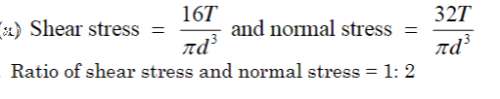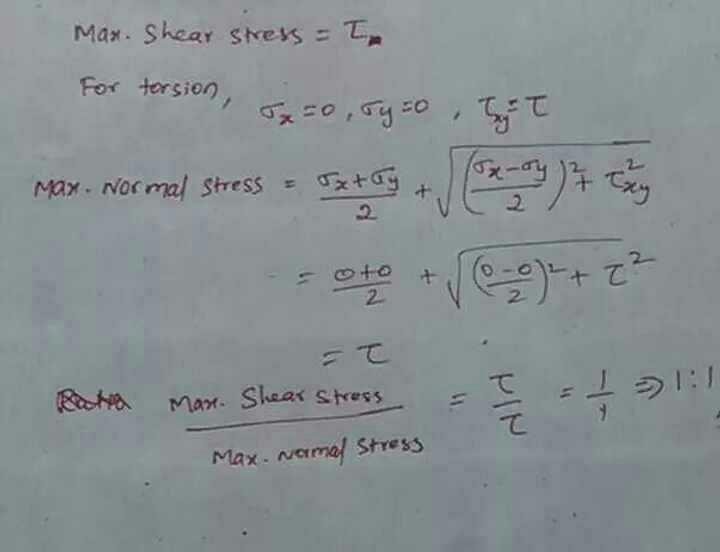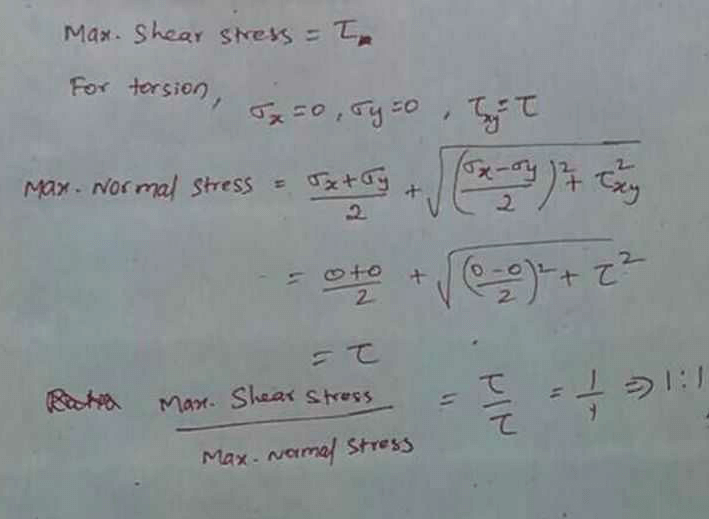Courses

# A solid circular shaft is subjected to pure torsion. The ratio of maximum shear stress to maximum normal stress at any point would bea)1 : 1b)1 : 2c)2 : 1d)2 : 3Correct answer is option 'A'. Can you explain this answer?

## Mechanical Engineering Question

Prince Singhania answered Jul 13, 2018Sidhant Kumar answered Jan 08, 2020Mohit Sharma answered Dec 19, 2018
Their will be the shear stress in the frame, hence Mohr circle will be around origin with radius of max. shear stress. Now, ratio of Max. Shear stress to Max. Normal stress is 1:1.

Vipin Kumar answered Jul 22, 2018
Ans a is correct because pour torsion is applied so shear stress is devloped when we draw the mohar circle we find maximum shear stress is equal to maximum normal stress.

neeraj singh answered Nov 05, 20191

Surendra Reddy answered Sep 24, 2018
A